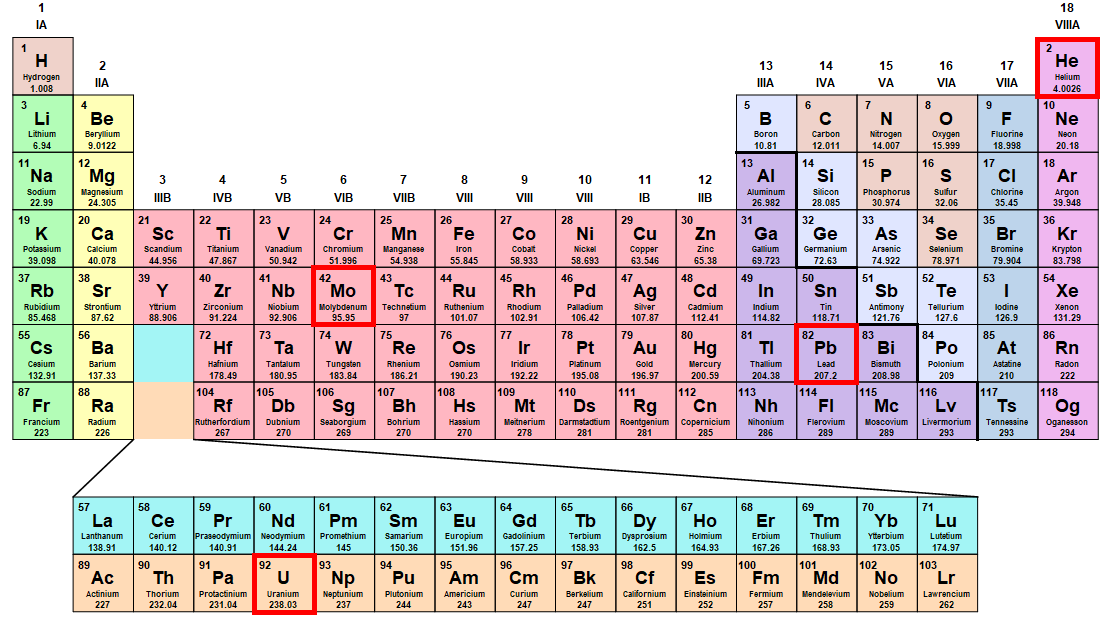# Very Small Physics 3

The founders of quantum mechanics largely ignored relativity when they first applied quantum theory to chemistry:

"The general theory of quantum mechanics is now almost complete... they give rise to difficulties only when high-speed particles are involved, and are therefore of no importance in the consideration of atomic and molecular structure and ordinary chemical reactions." - Paul Dirac, 1929

Treat an atom as a two-body problem with one electron and a central nucleus with a charge $Z$ and a mass much greater than the electron.

Was Dirac correct? At what element in the periodic table would relativistic effects become important for an electron in a ground state?Note: Significant errors start to appear between classical and relativistic dynamics when the Lorentz factor $\gamma = \frac{1}{\sqrt{1-v^2/c^2}} \geq 1.05$. It may be useful to perform this calculation in atomic units, where $\hbar, e, m_e,$ and $4 \pi \epsilon_0$ are defined as $1.$ In these units, the speed of light is $c = 137$.

×